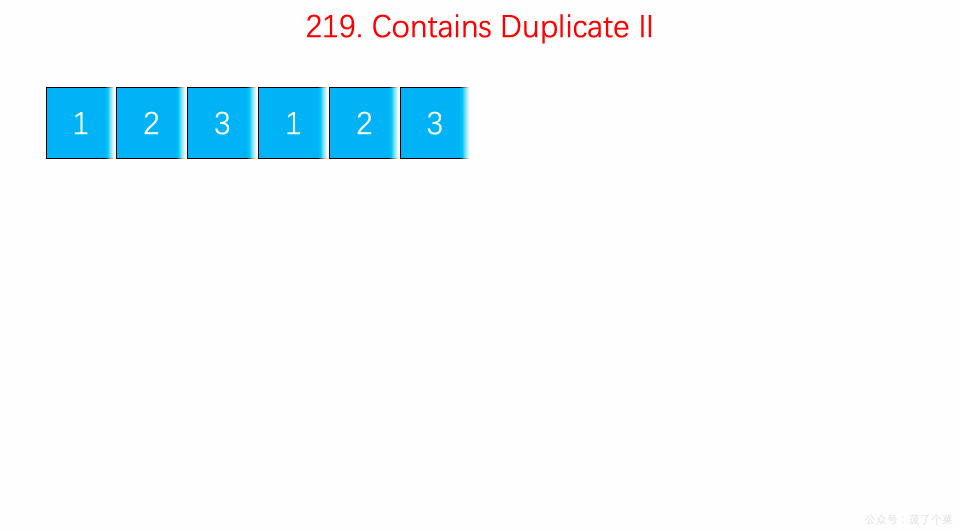# LeetCode 第 219 号问题：存在重复元素 II

### 题目描述

``````输入: nums = [1,2,3,1], k = 3

``````

``````输入: nums = [1,0,1,1], k = 1

``````

``````输入: nums = [1,2,3,1,2,3], k = 2

``````

### 题目解析

• 设置查找表`record`，用来保存每次遍历时插入的元素，`record`的最大长度为`k`
• 遍历数组`nums`，每次遍历的时候在`record`查找是否存在相同的元素，如果存在则返回`true`，遍历结束
• 如果此次遍历在`record`未查找到，则将该元素插入到`record`中，而后查看`record`的长度是否为`k + 1`
• 如果此时`record`的长度是否为`k + 1`，则删减`record`的元素，该元素的值为`nums[i - k]`
• 如果遍历完整个数组`nums`未查找到则返回`false`

### 动画描述### 代码实现

``````// 219. Contains Duplicate II
// https://leetcode.com/problems/contains-duplicate-ii/description/
// 时间复杂度: O(n)
// 空间复杂度: O(k)
class Solution {
public:
bool containsNearbyDuplicate(vector<int>& nums, int k) {

if(nums.size() <= 1)  return false;

if(k <= 0)  return false;

unordered_set<int> record;
for(int i = 0 ; i < nums.size() ; i ++){

if(record.find(nums[i]) != record.end()){
return true;
}

record.insert(nums[i]);

// 保持record中最多有k个元素
// 因为在下一次循环中会添加一个新元素,使得总共考虑k+1个元素
if(record.size() == k + 1){
record.erase(nums[i - k]);
}
}

return false;
}
};
``````